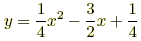index: click on a letter A B C D E F G H I J K L M N O P Q R S T U V W X Y Z A to Z index index: subject areas numbers & symbols sets, logic, proofs geometry algebra trigonometry advanced algebra & pre-calculus calculus advanced topics probability & statistics real world applications multimedia entrieswww.mathwords.com about mathwords website feedback

Vertex of a Parabola

The point at which a parabola makes its sharpest turn. The vertex is halfway between the directrix and the focus.Example:This is a graph of the parabolawith all its major features labeled: axis of symmetry, focus, vertex, and directrix.